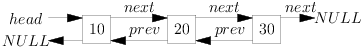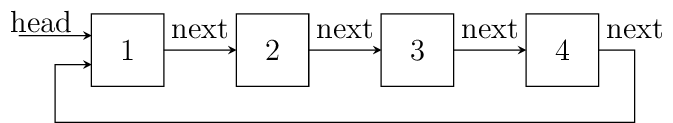# 7. Doubly Linked Lists and Circular Lists¶

In a singly linked list we have a next pointer using which we can move forward and iterate over the list. However, this severely restricts our movement in one direction although we can traverse back using recursion or a stack but that causes a loss in efficiency and is cumbersome. To avoid this situation we can have a doubly linked list which has an extra pointer typically called previous poiner with each node which points to the previous element. Thus first or head node’s previous pointer will point to NULL and next pointer of tail will point to NULL as usual.

Second advantage is that the delete operation is more efficient if the pointer to the node is given. Disadvantage is that for every node you need to more an extra pointer and all operations require maintenance of this extra pointer.## 7.2. dll.c¶

#include <stdio.h>
#include <stdlib.h>

int data;
}dll;

void append(dll**);
void insert_after(dll**);
void insert_before(dll**);
void print(dll*);
void search(dll*);
int count(dll*);

{
int value = 0;

if(q == NULL) {
printf("There is no node to be deleted.\n");
return;
}

printf("Enter the value to be deleted: ");
scanf("%d", &value);

if(q->data == value) { // case of deleting first node. head changes.
free(q);
return;
}

while(q->next != NULL) {
if(q->data == value) {
q->prev->next = q->next;
q->next->prev = q->prev;
free(q);
return;
}
temp = q;
q = q->next;
}

if(q->data == value) { // Last node is to be deleted
temp->next = NULL;
free(q);
return;
}

}

{
int count = 1;

printf("The no. of elements in linked list is %d.\n", 0);
return 0;
}

count++;
}

printf("The no. of elements in linked list is %d.\n", count);
return count;
}

{
puts("1. Add an element at beginning.");
puts("2. Add an element at after node at position x.");
puts("3. Add an element at before node at position x.");
puts("4. Add an element at end.");
puts("5. Count the number of elements.");
puts("6. Delete an element.");
puts("7. Search an element.");
puts("8. Print the list.");
}

{
int value = 0;
dll* node = (dll*)malloc(sizeof(dll));

printf("Enter a value for new node: ");
scanf("%d", &value);
node->data = value;
node->next = NULL; // because this is last node

node->prev = NULL;
return;
}

while(temp->next != NULL)
temp = temp->next;

temp->next = node;
node->prev = temp;
}

{
int position = -1;
int index = 0;
int value = 0;

printf("Enter the position at which node is to be inserted: ");
scanf("%d", &position);

if(position == 0) {
}

printf("Enter the value to be inserted: ");
scanf("%d", &value);

dll* temp = (dll*)malloc(sizeof(dll));
temp->data = value;

while(q->next != NULL) {
++index;
if(index == position){
temp->next = q->next;
temp->prev = q;
q->next->prev = temp;
q->next = temp;
return;
}
q = q->next;
}
++index;
if(index == position) { // q->next is NULL and j has matched position like append operation.
temp->next = NULL;
q->next = temp;
temp->prev = q;
return;
}
free(temp); // no match for position. free node else memory leak will happen.
}

{
int position = -1;
int index = 0;
int value = 0;

printf("We do not have any node to be inserted after.\n");
return;
}

printf("Enter the position at which node is to be inserted: ");
scanf("%d", &position);

printf("Enter the value to be inserted: ");
scanf("%d", &value);

dll* temp = (dll*)malloc(sizeof(dll));
temp->data = value;

while(q->next != NULL) {
if(index == position){
temp->next = q->next;
temp->prev = q;
q->next->prev = temp;
q->next = temp;
return;
}
q = q->next;
++index;
}

if(q->next == NULL && index == position) { // equivalent to append
temp->next = q->next;
temp->prev = q;
q->next = temp;
return;
}
}

{
dll* temp = (dll*)malloc(sizeof(dll));
int value = 0;

printf("Enter a value for new node: ");
scanf("%d", &value);

temp->data = value;
temp->prev = NULL;

}

{
}

printf("NULL\n");
}

{
int i=0, position=1;

printf("Enter the number to be searched.");
scanf("%d", &i);

printf("%d is found at %dth position.\n", i, position - 1);
return;
}

position++;
}
}

int main()
{
int option = 0;

printf("Enter 1 to 8 to choose an action. Any other value to quit.\n");
scanf("%d", &option);
getchar(); // to remove \n

while(option  >= 1 && option <= 8) {
switch(option) {
case 1:
break;
case 2:
break;
case 3:
break;
case 4:
break;
case 5:
break;
case 6:
break;
case 7:
break;
case 8:
break;
default:
break;
}
printf("Enter 1 to 8 to choose an action. Any other number to quit.\n");
fflush(stdin);
scanf("%d", &option);
getchar(); // to remove \n
}

return 0;
}


You can extrapolate the animations of singly linked list to doubly linked list as well just that you have to take care of prev pointer as well.

## 7.3. Circular Lists¶

Circular list is a singly linked list where last node’s next pointer is not NULL but rather it points to head of the list. Let us try to reimplement all the functions we had implemented for singly linked list. The only difference is that we will point last nodes next pointer to head. I have said it is a singly linked list but a doubly linked list can also have a circular representation which we will discuss once we are through with this.

Fundamentally, it should look like following(assume that data contained in each node is an integer):Circular list representation.

1. Any node can be head i.e. starting point. We can always traverse the entire list using this node. Clearly, we will need to stop when next pointer of any node becomes points to this node.
2. Certain problems are solved more easily using circular lists, for example, in the linked list chapter the problem on arbitrary precision integers can be solved much more easily using circular lists. Fibonacci heap is another example where queues are used.
3. Queues can be implemented using circular lists. We do not need to maintain rear pointer if we have doubly circular linked list which will allow us to move in both directions.
4. Real world queues can be simulated using circular linked lists which is obvious from point 2.

Given below is complete implementation of singly cicular linked list:

#include <stdio.h>
#include <stdlib.h>

int data;
} cll;

void print(cll*);
void search(cll*);
void delete(cll**);
void append(cll**);

{
cll *temp, *q;
int i;

printf("There is no element to be deleted.\n");
return;
}

printf("Enter the value of data to be deleted.\n");
scanf("%d", &i);

if(temp->data == i) {
if(temp == temp->next) { // there is only one node
free(temp);
return;
}

cll *to_delete = temp;
temp = temp->next;
temp->next = temp->next->next;
free(to_delete);
return;
} else {
if(temp->data == i) {
q->next = temp->next;
free(temp);
return;
}
q = temp;
temp = temp->next;
}
}

if(temp->data == i) { // case of deletion of last node
q->next = temp->next;
free(temp);
return;
}

}

{
int count = 1;

printf("The no. of elements in linked list is %d.\n", 0);
return 0;
}

count++;
}

printf("The no. of elements in linked list is %d.\n", count);
return count;
}

{
puts("1. Add an element at beginning.");
puts("2. Add an element at position n.");
puts("3. Add an element at end.");
puts("4. Count the number of elements.");
puts("5. Delete an element.");
puts("6. Search an element.");
puts("7. Print the list.");
}

{
int i;

printf("Enter the number which is to be appended to the list.\n");
scanf("%d", &i);

temp = (cll*)malloc(sizeof(cll));
temp->data = i;
temp->next = NULL;

temp->next = temp;
return;
}

q = q->next;
}

q->next = temp;
}

{
int i = 0, j = 0;
int position = 0;

printf("Enter position at which the number is to be added.\n");
scanf("%d", &position);

if(position == 0)

temp = (cll*)malloc(sizeof(cll));

printf("Enter an integer to be added in between.\n");
scanf("%d", &i);

++j;
if(j == position) {
temp->next = q->next;
q->next = temp;
temp->data = i;
return;
}
q = q->next;
}
++j;
// This is the case when q->next is *head so it is an append
// operation
if(j == position) {
}
free(temp); // no insertion happened so we need to free temp
// i.e. j was out of possible positions
}

{
int i;
cll *it = NULL; // iterator pointer

it = (cll*)malloc(sizeof(cll));

printf("Enter an integer to be added at beginning\n");
scanf("%d", &i);

it->data = i;

temp = temp->next;
temp->next = it;
} else {
it->next = it;
}
}

{

return;

do {

}

{
int i=0, position=1;

return;

printf("Enter the number to be searched.");
scanf("%d", &i);

do {
printf("%d is found at position %dth\n", i, position);
return;
}
position++;
}

int main()
{
int option = 0;

printf("Enter 1 to 7 to choose an action. Any other number to quit.\n");
scanf("%d", &option);
getchar(); // to remove \n

while(option  >= 1 && option <= 7) {
switch(option) {
case 1:
break;
case 2:
break;
case 3:
break;
case 4:
break;
case 5:
break;
case 6:
break;
case 7:
break;
default:
break;
}